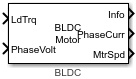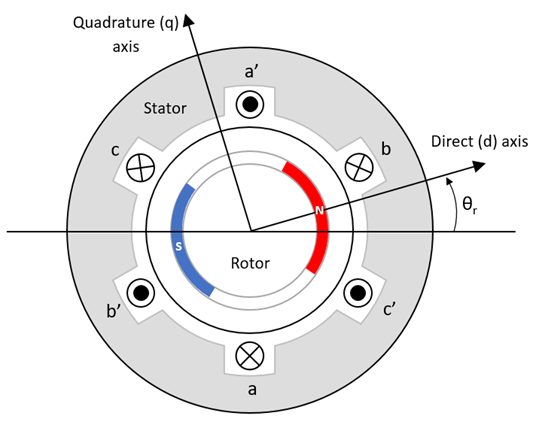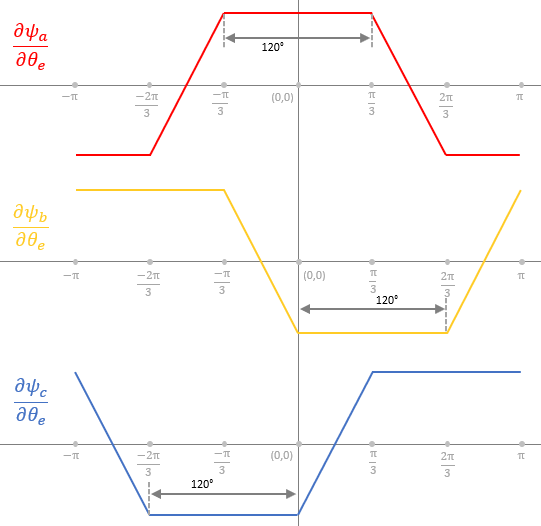# BLDC

Three-phase brushless DC motor with trapezoidal flux distribution

Since R2023a

•Libraries:
Motor Control Blockset / Electrical Systems / Motors

## Description

The BLDC block implements a three-phase brushless DC (BLDC) motor with a trapezoidal back electromotive force that remains constant for a position range of 120 electrical degrees. The block uses the three-phase input voltages to regulate the individual phase currents, allowing control of the motor torque or speed.

By default, the block sets the Simulation type parameter to `Continuous` to use a continuous sample time during simulation. If you want to use the block for fixed-step simulation, set the parameter to `Discrete`. Then specify a Sample time (s) parameter.

### Motor Construction

This figure shows a motor with a single pole pair.The motor magnetic field due to the permanent magnets creates a trapezoidal rate of change of flux with the motor angle.

For the axes convention, the a-phase and permanent magnet fluxes align when the motor angle θr is zero.

### Three-Phase Sinusoidal Model Electrical System

Instead of the standard dq reference frame, the block uses d’q’ reference frame, which is defined by these transformation equations.

`${v}_{d\text{'}}=\frac{2}{3}\left(\left({v}_{a}-R{i}_{a}-{E}_{a}\right)\mathrm{cos}{\theta }_{e}+\left({v}_{b}-R{i}_{b}-{E}_{b}\right)\mathrm{cos}\left({\theta }_{e}-\frac{2\pi }{3}\right)+\left({v}_{c}-R{i}_{c}-{E}_{c}\right)\mathrm{cos}\left({\theta }_{e}+\frac{2\pi }{3}\right)\right)$`

`${v}_{q\text{'}}=-\frac{2\pi }{3}\left(\left({v}_{a}-R{i}_{a}-{E}_{a}\right)\mathrm{sin}{\theta }_{e}+\left({v}_{b}-R{i}_{b}-{E}_{b}\right)\mathrm{sin}\left({\theta }_{e}-\frac{2\pi }{3}\right)+\left({v}_{c}-R{i}_{c}-{E}_{c}\right)\mathrm{sin}\left({\theta }_{e}+\frac{2\pi }{3}\right)\right)$`

`$\begin{array}{l}{E}_{a}=P{\omega }_{m}\frac{\partial {\psi }_{a}}{\partial {\theta }_{e}}\\ {E}_{b}=P{\omega }_{m}\frac{\partial {\psi }_{b}}{\partial {\theta }_{e}}\\ {E}_{c}=P{\omega }_{m}\frac{\partial {\psi }_{c}}{\partial {\theta }_{e}}\end{array}$`

`$\begin{array}{l}{i}_{a}={i}_{d\text{'}}\mathrm{cos}{\theta }_{e}-{i}_{q\text{'}}\mathrm{sin}{\theta }_{e}\\ {i}_{b}={i}_{d\text{'}}\mathrm{cos}\left({\theta }_{e}-\frac{2\pi }{3}\right)-{i}_{q\text{'}}\mathrm{sin}\left({\theta }_{e}-\frac{2\pi }{3}\right)\\ {i}_{c}={i}_{d\text{'}}\mathrm{cos}\left({\theta }_{e}+\frac{2\pi }{3}\right)-{i}_{q\text{'}}\mathrm{sin}\left({\theta }_{e}+\frac{2\pi }{3}\right)\end{array}$`

The block implements these equations, expressed in the dq and d’q’ reference frame of the BLDC motor. All quantities in the motor reference frame are with respect to the stator phase A.

`$\begin{array}{l}{\omega }_{e}=P{\omega }_{m}\\ \frac{d}{dt}{i}_{d\text{'}}=\frac{1}{{L}_{d}}{v}_{d\text{'}}+\frac{{L}_{q}}{{L}_{d}}P{\omega }_{m}{i}_{q\text{'}}\end{array}$`

`$\frac{d}{dt}{i}_{q\text{'}}=\frac{1}{{L}_{q}}{v}_{q\text{'}}-\frac{{L}_{d}}{{L}_{q}}P{\omega }_{m}{i}_{d\text{'}}$`

`${T}_{e}=1.5P\left(\left({L}_{d}-{L}_{q}\right){i}_{d\text{'}}{i}_{q\text{'}}\right)+P\left(\frac{\partial {\psi }_{a}}{\partial {\theta }_{e}}{i}_{a}+\frac{\partial {\psi }_{b}}{\partial {\theta }_{e}}{i}_{b}+\frac{\partial {\psi }_{c}}{\partial {\theta }_{e}}{i}_{c}\right)$`

This table describes the variables used in these equations.

 Lq, Ld q- and d-axis inductances (H) R Resistance of the stator windings (ohm) iq', id' q'- and d'-axis currents (A) vq', vd' q'- and d'-axis voltages (V) ωm Angular mechanical velocity of the motor (rad/s) ωe Angular electrical velocity of the motor (rad/s) P Number of pole pairs Te Electromagnetic torque (Nm) Θe Electrical angle (rad) Va, Vb, Vc Stator phase A, B, and C voltages (V) ia, ib, ic Stator phase A, B, and C currents (A) Ea, Eb, Ec Back EMF of stator phases A, B, and C (V/m/s) Ψa, Ψb, Ψc Total fluxes linking each stator winding (Wb)

### Mechanical System

The motor angular velocity is given by:

`$\begin{array}{c}\frac{d}{dt}{\omega }_{m}=\frac{1}{J}\left({T}_{e}-{T}_{f}-F{\omega }_{m}-{T}_{m}\right)\\ \frac{d{\theta }_{m}}{dt}={\omega }_{m}\end{array}$`

The equations use these variables.

 J Combined inertia of motor and load (kgm^2) F Combined viscous friction of motor and load (N·m/(rad/s)) θm Motor mechanical angular position (rad) Tm Motor shaft torque (Nm) Te Electromagnetic torque (Nm) Tf Motor shaft static friction torque (Nm) ωm Angular mechanical velocity of the motor (rad/s)

### Trapezoidal Rate of Change of Flux

The rotor magnetic field due to the permanent magnets create a trapezoidal rate of change of flux with the rotor angle. This figure shows the rate of change of flux for the three phases of a three-phase BLDC motor.## Ports

### Input

expand all

Load torque on the motor shaft, Tm, in N·m.

#### Dependencies

To enable this port, set the Mechanical input configuration parameter to `Torque`.

Angular velocity of the motor, ωm, in rad/s.

#### Dependencies

To enable this port, set the Mechanical input configuration parameter to `Speed`.

Stator terminal voltages, Va, Vb, and Vc, in V.

### Output

expand all

The bus signal contains these block calculations.

Signal DescriptionVariableUnits

`IaStator`

Stator phase current A

ia

A

`IbStator`

Stator phase current B

ib

A

`IcStator`

Stator phase current C

ic

A

`IdSync`

d' axis current

id'

A

`IqSync`

q' axis current

iq'

A

`VdSync`

d' axis voltage

vd'

V

`VqSync`

q' axis voltage

vq'

V

`MtrSpd`

Angular mechanical velocity of the motor

ωm

`MtrPos`

Motor mechanical angular position

θm

`MtrTrq`

Electromagnetic torque

Te

N·m

`MtrHall`

Hall sensor output

--

`BackEMF`

Back EMF generated in motorEa, Eb, Ec

V/m/s

Phase a, b, c current, ia, ib, and ic, in A.

Motor torque, Tmtr, in N·m.

#### Dependencies

To enable this port, set the Mechanical input configuration parameter to `Speed`.

Angular speed of the motor, ωmtr, in rad/s.

#### Dependencies

To enable this port, set the Mechanical input configuration parameter to `Torque`.

## Parameters

expand all

### Block Options

This table summarizes the port configurations.

Port ConfigurationCreates Input PortCreates Output Port

`Torque`

`LdTrq`

`MtrSpd`

`Speed`

`Spd`

`MtrTrq`

By default, the block uses a continuous sample time during simulation. If you want to generate code for single-precision targets, set the parameter to `Discrete`.

Integration sample time for discrete simulation, in s.

#### Dependencies

To enable this parameter, set Simulation type to `Discrete`.

### Parameters

Motor pole pairs, P.

Stator resistance per phase, Rs, in ohms.

Stator inductance across d-axis, Ld, in H.

Stator inductance across q-axis, Lq, in H.

Permanent flux linkage constant, λpm, in Wb.

Rotor inertia, J, in kg.m^2.

Static friction, Tf, in N·m.

### Initial Values

Initial d-axis current, id', in A.

Initial q-axis current, iq', in A.

Initial angular position of motor, θm0, in rad.

Initial angular velocity of the motor, ωm0, in rad/s.

#### Dependencies

To enable this parameter, set Mechanical input configuration to `Torque`.

## Version History

Introduced in R2023a